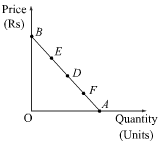### Write short notes on: 1. Geometric method

Write short notes on:

1. Geometric method

Answer: Geometric method is also called point method of measuring elasticity. Under this method, elasticity is measured at different points on a demand curve. This method of measuring price elasticity was developed by Dr. Marshall. A demand curve can either be a straight line demand curve or a rectangular hyperbola curve. Accordingly, we study the geometric method of measuring elasticity for the two types of demand curves. The price elasticity on any point of the demand curve is calculated by using the following formula.In the figure, AB is a straight line demand curve
Now consider point D on the demand curve. Elasticity at this point (D) can be calculated as:As the point D lies at the middle of the demand curve, so the lower segment of point (i.e. DA) is equal to the upper segment of point (i.e. DB). Thus, ed at point D is equal to 1.
At point B,  point elasticity of demand is equal toAs against this, at point A, the elasticity of demand is equal toAt point F, the elasticity of demand is calculated as: